ING styles for common plotting libraries

# ing-theme-matplotlib

ing_theme_matplotlib is a python package with a light and a dark matplotlib and seaborn style that allows you to create your plots using ING colors and ING Me font. It was adapted from the qbstyles package.

Dark style Light style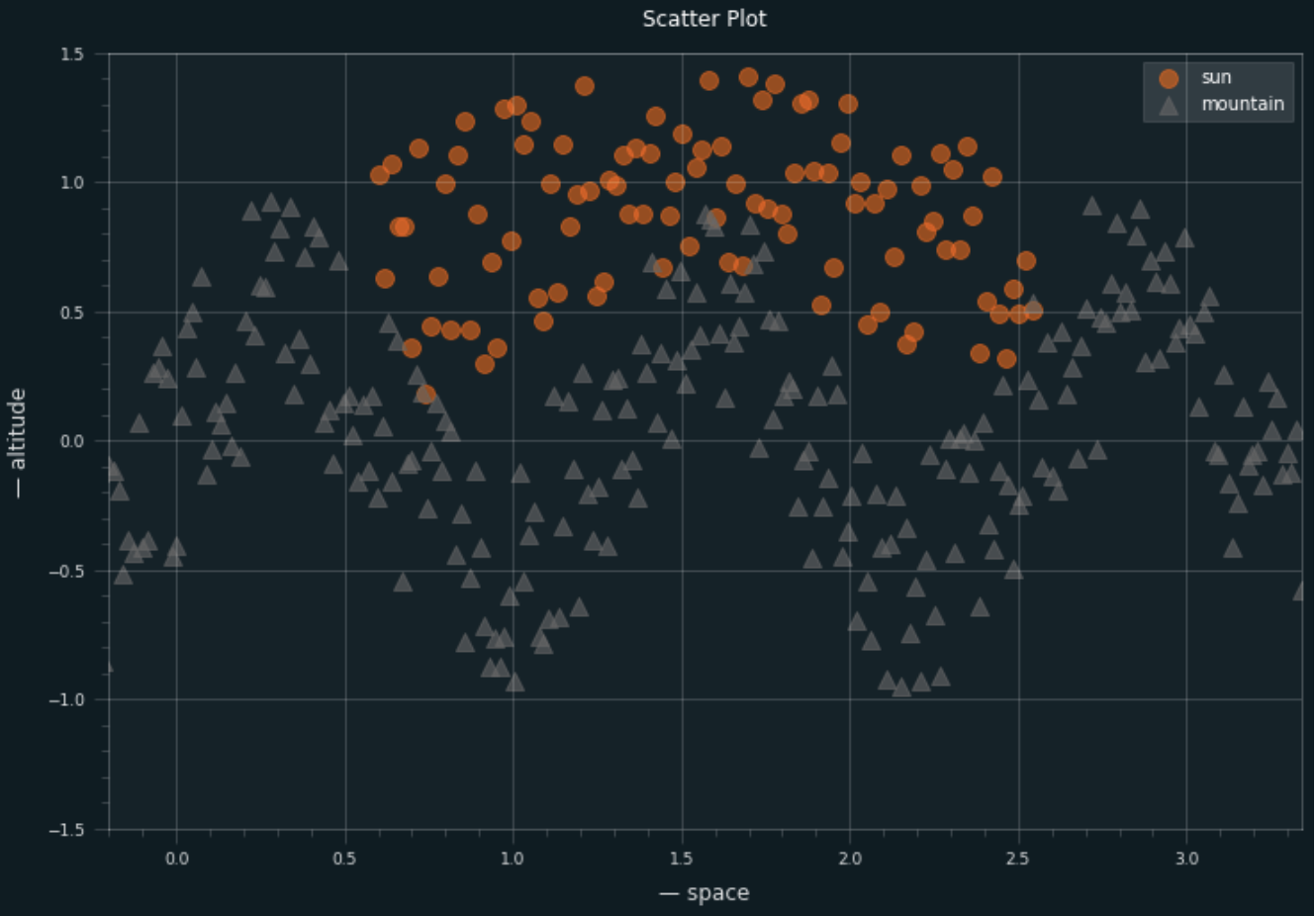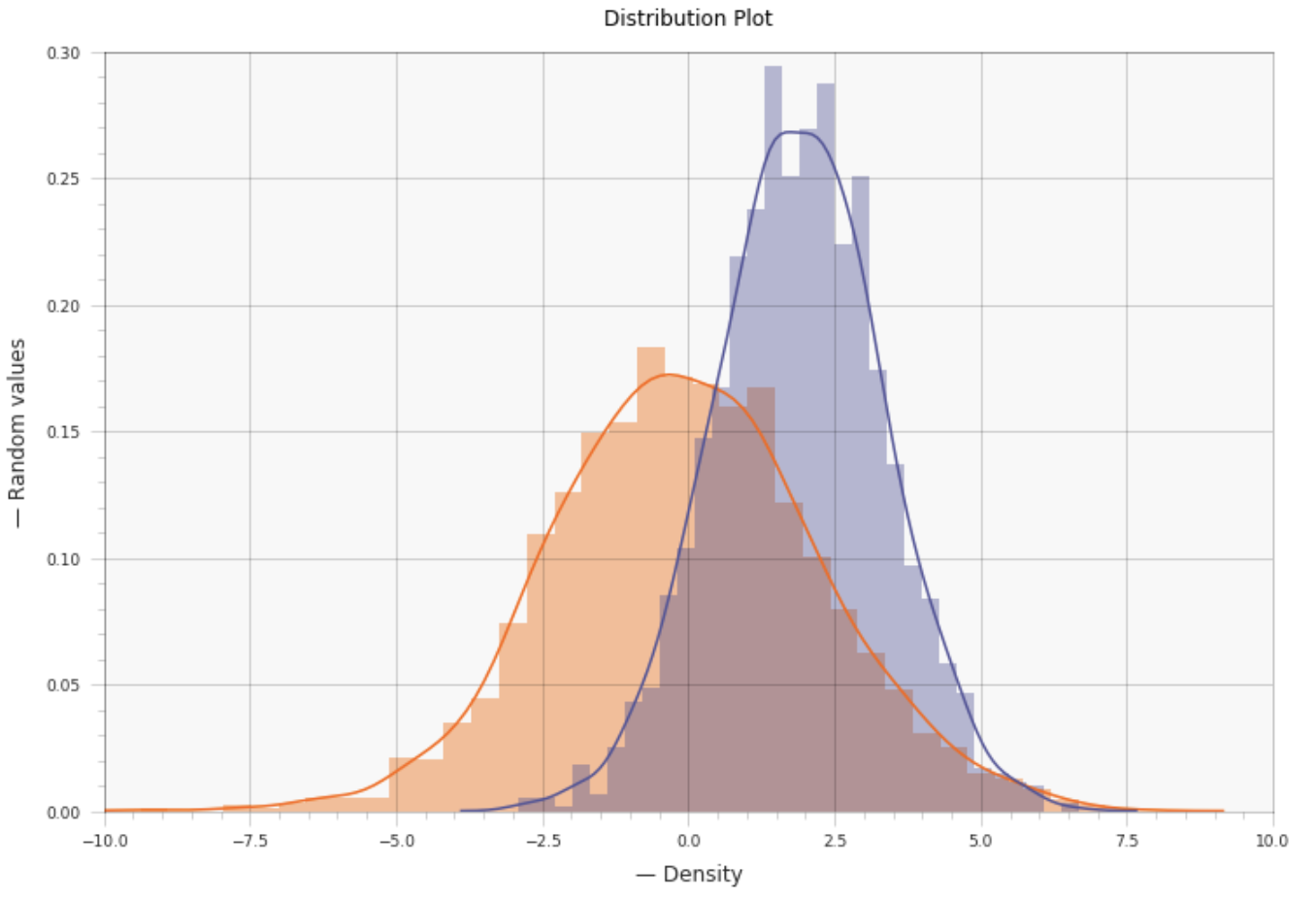## Installation

pip install ing_theme_matplotlib


## Usage

You can use the dark Matplotlib style theme in the following way:

from ing_theme_matplotlib import mpl_style

mpl_style(dark=True)


And to use the light Matplotlib style theme, you can do the following:

from ing_theme_matplotlib import mpl_style

mpl_style(dark=False)


⚠️ Make sure to run from ing_theme_matplotlib import mpl_style and mpl_style() in different cells as shown above. See this issue.

Assume that below is the function we use for plotting;

def line_plot(ax):
rng = np.random.RandomState(4)
x = np.linspace(0, 10, 500)
y = np.cumsum(rng.randn(500, 4), 0)
ax.set_title('Line Graph')
ax.set_xlabel('— Time')
ax.set_ylabel('— Random values')
ax.plot(x, y, label = ['Bitcoin', 'Ethereum', 'Dollar', 'Oil'])
ax.legend(['Bitcoin', 'Ethereum', 'Dollar', 'Oil'], loc = 1, fontsize = 'medium')
ax.set_xlim([0, 10])
ax.set_ylim([-20, 60])
ax.figure.set_figwidth(16)
ax.figure.set_figheight(8)
ax.spines['right'].set_position(('axes', 1.05))
ax.spines['right'].set_color('none')


You can add the default ing logo to your plot by calling add_logo function inside the plotting function.

from ing_theme_matplotlib.mpl_style import add_logo

mpl_style()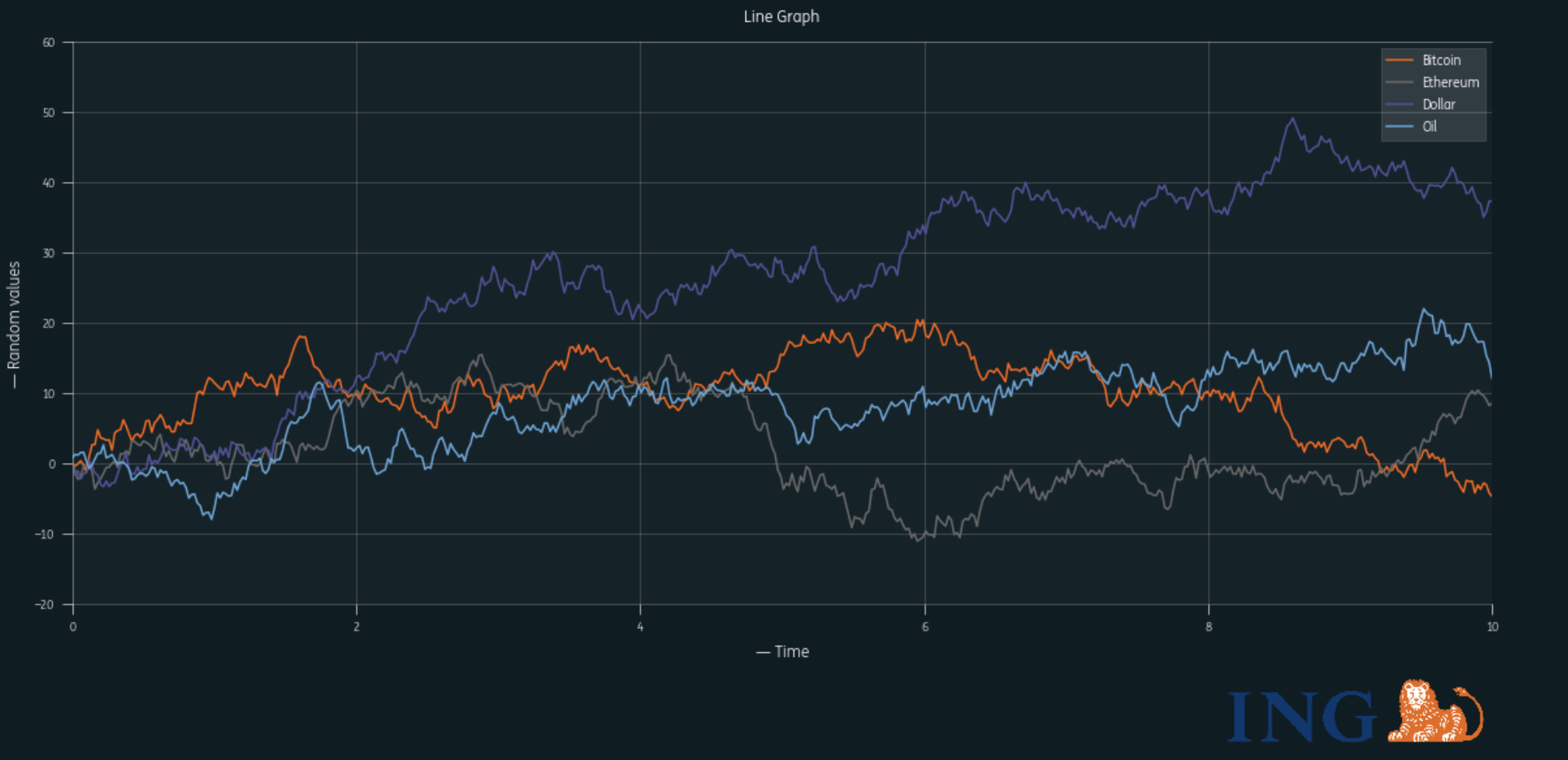You can also add custom logos to your plot by giving the path where the image is located.

from ing_theme_matplotlib.mpl_style import add_logo

mpl_style(dark = False)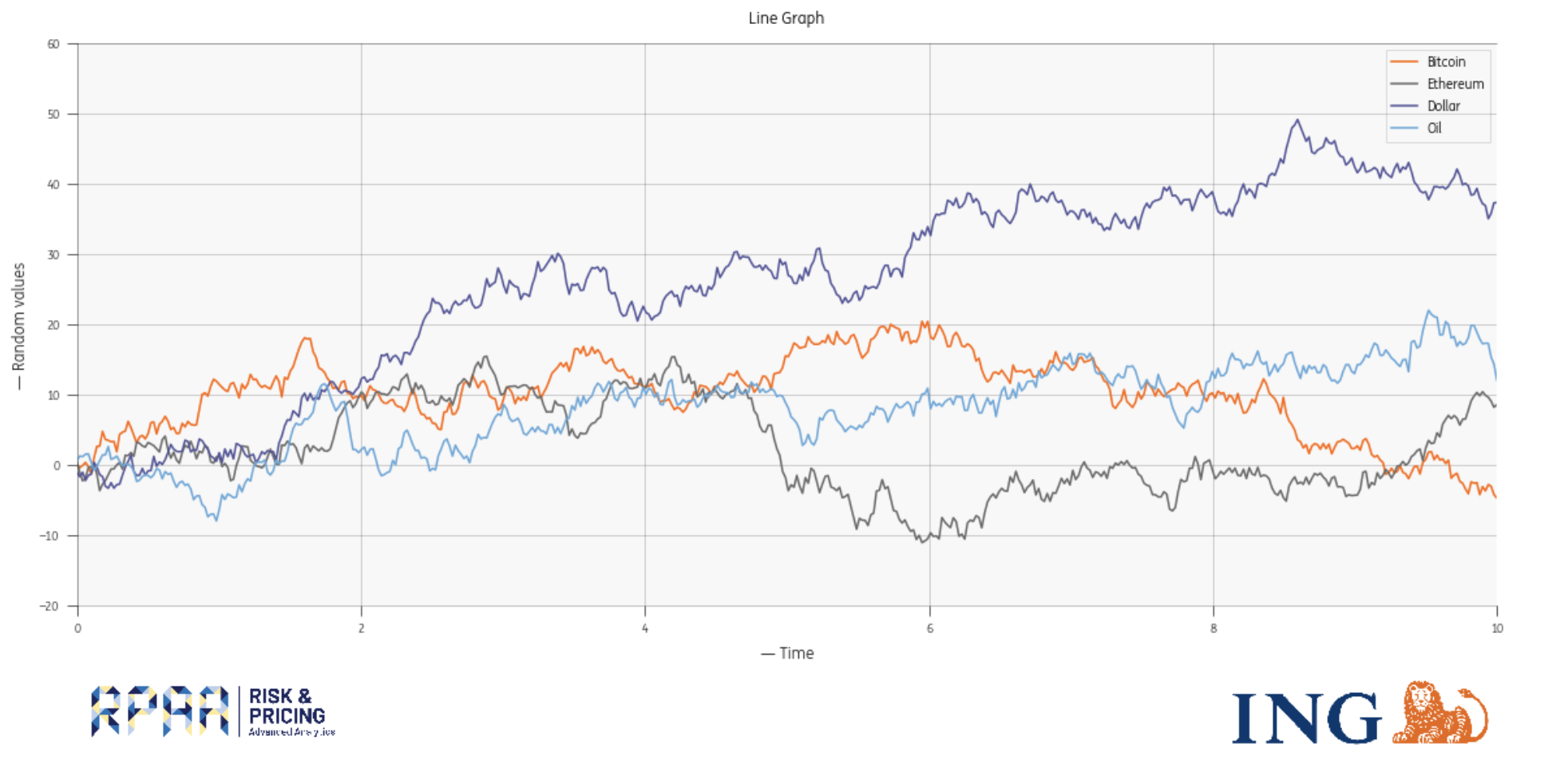For more examples see ExamplePlots.ipynb.

## Seaborn Usage

Similar to above, you can implement plots in Seaborn. The main difference is how the logo is added.

mpl_style(dark = False)
def bar_plot():
ax = sns.barplot(x="tip", y="day", data=tips, ax=logo.axes)

bar_plot()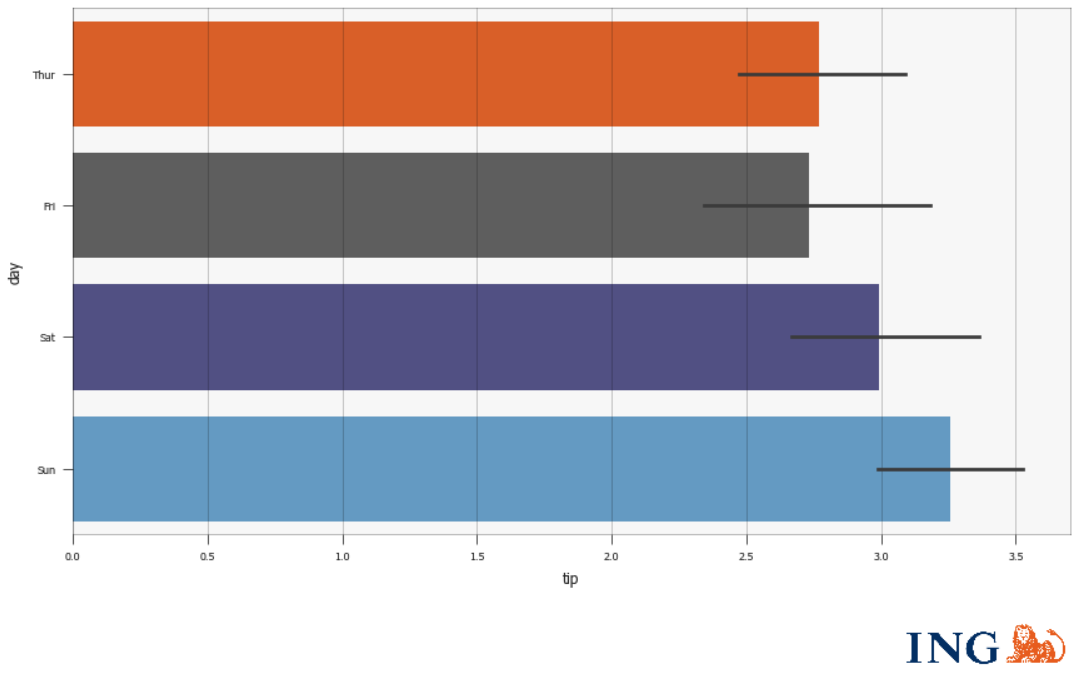For more examples see [Example Seaborn Plots.ipynb](Example Seaborn Plots.ipynb).

## Supported chart types

• Line plots
• Scatter plots
• Bubble plots
• Bar charts
• Pie charts
• Histograms and distribution plots
• 3D surface plots
• Stream plots
• Polar plots

## plt.Figure()

It is important to note that we use plt.Figure_ING() in place of plt.Figure() due to changes in Matplotlib version 3.4.1. As plt.subplots() works smoothly, it is best to stick to that methodology.

## What licence do we use?

ING Style plotting is licensed under the Apache 2.0 License. ing-theme-matplotlib is forked from qbstyles.

## Project details

This version0.1.80.1.70.1.60.1.50.1.40.1.30.1.20.1.10.1.0

Uploaded source